New variable separation solutions for the generalized nonlinear diffusion equations
School of Science, Xi’an University of Architecture and Technology, Xi’an 710055, China
Center for Nonlinear Studies, School of Mathematics, Northwest University, Xi’an 710069, China

† Corresponding author. E-mail: feiyuji@xauat.edu.cn

Project supported by the National Natural Science Foundation of China (Grant Nos. 11371293, 11401458, and 11501438), the National Natural Science Foundation of China, Tian Yuan Special Foundation (Grant No. 11426169), and the Natural Science Basic Research Plan in Shaanxi Province of China (Grant No. 2015JQ1014).

Abstract
Abstract

The functionally generalized variable separation of the generalized nonlinear diffusion equations ut = A(u,ux)uxx + B(u,ux) is studied by using the conditional Lie–Bäcklund symmetry method. The variant forms of the considered equations, which admit the corresponding conditional Lie–Bäcklund symmetries, are characterized. To construct functionally generalized separable solutions, several concrete examples defined on the exponential and trigonometric invariant subspaces are provided.

1. Introduction

It is well known that symmetry-related methods play important roles in studying symmetry reductions and constructing exact solutions to nonlinear partial differential equations (NPDEs). Up to now, a number of effective approaches have been presented. For instance, the Lie point symmetry method, also known as the classical symmetry method due to Sophus Lie in 1881, is regarded as the most popular technique. The nonclassical symmetry method, as an extension of Lie’s classical method, which was introduced by Bluman and Cole, can be applied to find more types of exact solutions to some NPDEs. In comparison with the symmetry method, the direct method proposed by Clarkson and Kruskal can be used to realize reductions of NPDEs without any reference to the Lie group theory. Here we especially emphasize the conditional Lie–Bäcklund symmetry (CLBS) method introduced by Fokas and Liu and Zhdanov, which is a natural extension of the nonclassical symmetry method. For that matter, the algorithm of the CLBS is similar to that of the nonclassical symmetry. From the point of view of the ansatz form of the solution, we now focus on the following two kinds of ansatzs. One is the functional separable solution (FSS)[20,21]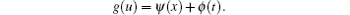The other is the derivative-dependent functional separable solution[22,23]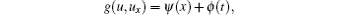which is an extension of the former. These two variable separation approaches based on their corresponding CLBSs have been widely applied to classify and construct exact solutions of some nonlinear evolution equations.

In this paper, we develop the CLBS method to study the functionally generalized separable solutions (FGSSs)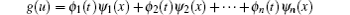to the generalized nonlinear diffusion equations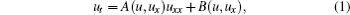where A(u,ux) and B(u,ux) are smooth functions of the indicated variables to be settled. Obviously, the FGSS is an extension of the FSS. The FSSs to Eq. (1) were solved by using CLBS. Furthermore, seeking for the approximate FSSs of Eq. (1) with perturbation was taken by way of approximate CLBS.

The layout of this paper is as follows. In Section 2, we review some basic definitions and fundamental theorems on the CLBS method and the invariant subspace (IS) method. In Section 3, the classification of Eq. (1) admitting certain CLBSs and the corresponding ISs are presented. In Section 4, some FGSSs to the resulting equations are constructed by way of examples. The last section gives the conclusion and discussion.

2. Preliminaries

For convenience, we review some basic facts on the CLBS and the IS methods for the nonlinear evolution equations.

Consider the k-th order nonlinear evolution equation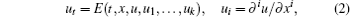letbe an evolutionary vector field and η = η(t,x,u,u1,u2,…,un) be its characteristic, where the total derivative operators are defined as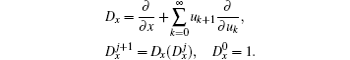Definition 1 The evolutionary vector field (3) is said to be a Lie–Bäcklund symmetry of Eq. (2) if and only if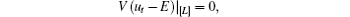where [L] is the set of all the differential consequences of Eq. (2), that is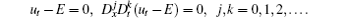Definition 2 The evolutionary vector field (3) is said to be a CLBS of Eq. (2) if and only if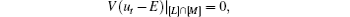where [M] denotes the set of all the differential consequences of equation η = 0 with respect to x, that is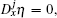j = 0,1,2,….

Proposition 1[18,19] Equation (2) admits the CLBS (3) if there exists a function S(t,x,u,η) so that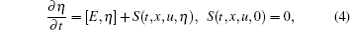where [E,η] = E′ηη′E, the prime denotes the Fréchet derivative, and S is an analytic function of t, x, u, ux,…and η, Dxη,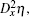….

Following this proposition, equation (2) admits the CLBS (3) with the characteristic η if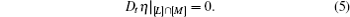Letbe a characteristic of Eq. (1), where [g(u)]ix = i(g(u))/∂xi.

If equation (1) admits CLBS (6), then equation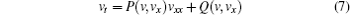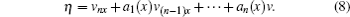In fact, equation (1) is related to Eq. (7) via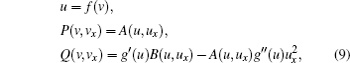where the primes denote the derivatives with respect to u, and u = f(v) denotes the inverse function of v = g(u). To proceed, the following proposition is necessary.

Proposition 2 Equation (7) admits the CLBS with the characteristic (8) if and only if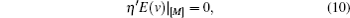where the prime denotes the Fréchet derivative, and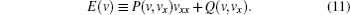By Proposition 2, we conclude that equation (10) is right, the invariance condition of the subspace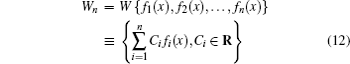with respect to the operator E, where Wn is the solution space of η = vnx + a1(x)v(n−1)x + … + an(x)v = 0.

Thus it is easy to know that E[Wn] ⊂ Wn, that is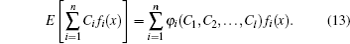If equation (7) admits CLBS (8), then equation (7) has the generalized separable solution (GSS)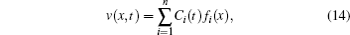where the coefficients {Ci(t)} are determined by the first order dynamical system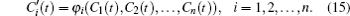To estimate the dimension of the IS admitted by the operator E, the following theorem is helpful.

Theorem 1 If linear space Wn is invariant with respect to the nonlinear operator E of order j, then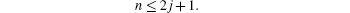In terms of this theorem, we investigate CLBSs (8) with 2 ≤ n ≤ 5 for Eq. (7).

3. Equation (7) admitting GSSs (14)

We first consider the case of n = 2.

By proposition 2, equation (7) admits CLBS (8) if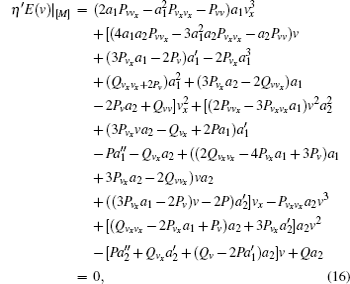where P = P(v,vx), Q = Q(v,vx), and ai = ai(x) (i = 1,2). The primes denote the derivatives with respect to x, and the subscripts denote the partial derivatives with respect to the indicated variables.

Because not all solutions of Eq. (16) can be obtained, we just present some special solutions listed in Table 1. It is worth noting that the following cases: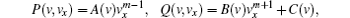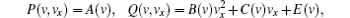are two special cases in Eq. (7).Table 1.

CLBSs (8) and ISs with n = 2 of Eq. (7).

.

In Table 1,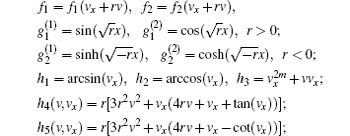and r, s, m, and n are arbitrary constants in their sets of definition when not specified.

In the same way, we can obtain the determining systems for n = 3,4,5. Since the determining systems are quite complicated, they are omitted here. Finally, a complete classification to CLBSs (8) and ISs (12) with n = 3,4,5 of Eq. (7) is presented in Table 2.

In Table 2,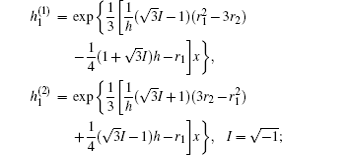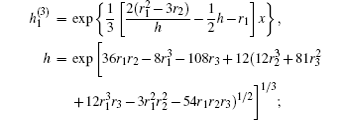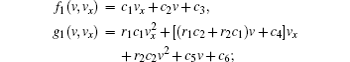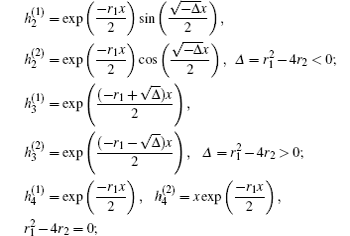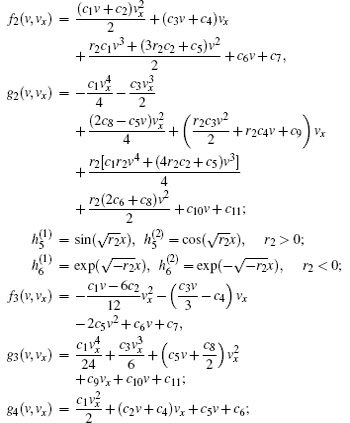and ri, ci (i ∈ N) are arbitrary constants in their sets of definition when not specified.Table 2.

CLBSs (8) and ISs with n = 3,4,5 of Eq. (7).

.
4. Some GSSs of Eqs. (1) and (7)

In this section, we construct GSSs to the equations obtained in the last section. These solutions are determined explicitly with some finite-dimensional dynamical systems. We take several examples to illustrate the calculation procedure.

Example 1 The equation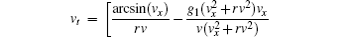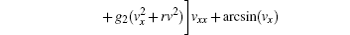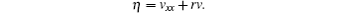By the transformation v = g(u), we can obtain the corresponding FGSS of the equation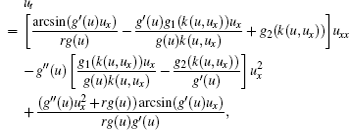where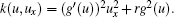Example 2 The equation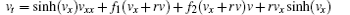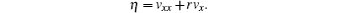The corresponding GSS is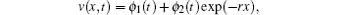where ϕ1(t) and ϕ2(t) satisfy the following dynamical system: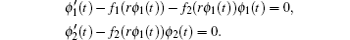By the appropriate transformation v = g(u), we can obtain the FGSS of the equation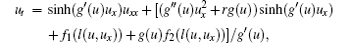where l(u,ux) = g′(u)ux + rg(u).

Example 3 The equation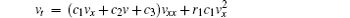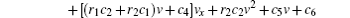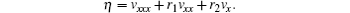The corresponding GSSs are given as follows.

By the appropriate transformation v = g(u), we can obtain the FGSSs of the equation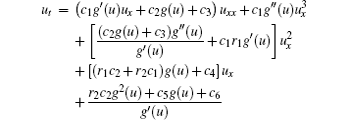from the above GSSs of Eqs. (17)–(19) respectively.

Example 4 The equation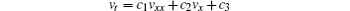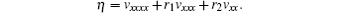The corresponding GSSs are given below.

By the appropriate transformation v = g(u), we can respectively obtain the FGSSs of the equation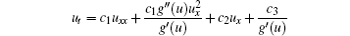from the above GSSs of Eqs. (20)–(22).

5. Discussion and conclusion

By using the CLBS and IS methods, we have studied the problem of FGSSs for the generalized nonlinear diffusion equations. Those equations admitting the corresponding CLBSs with ISs have been obtained and displayed in tables. Finally, we have derived the FGSSs of some resulting equations. In general, these solutions cannot be obtained by other relevant approaches.

There are still two interesting questions to be answered in the future: (i) How to develop the CLBS method with IS to investigate the FGSSs of other types of evolution equations, such as higher-dimensional equations? (ii) How to solve the approximate FGSS of NPDEs with perturbation terms? We expect that certain other types of NPDEs could be investigated by means of the CLBS and the IS approaches, and some new results would be obtained sooner or later.

Reference
 1 Bluman G WKumei S1989Symmetries and Differential EquationsNew YorkSpringer-Verlag 2 Olver P J1993Applications of Lie Groups to Differential EquationsNew YorkSpringer-Verlag 3 Ibragimov N H1985Transformation Groups Applied to Mathematical PhysicsBostonReidel 4 Ovsiannikov L V1982Group Analysis of Differential EquationsNew YorkAcademic Press 5 Bluman G WCole J D1969J. Math. Mech.181025 6 Ames W F1972Nonlinear Partial Differential Equations in EngineeringNew YorkAcademic Press 7 Levi DWinternitz P 1989 J. Phys. A: Math. Gen. 22 2915 8 Wang J YTang X YLiang Z FLou S Y 2015 Chin. Phys. B 24 050202 9 Hu X RChen Y 2015 Chin. Phys. B 24 090203 10 Liu X ZYu JR B 2015 Chin. Phys. B 24 030202 11 Wang XMa T BNing J G 2014 Chin. Phys. Lett. 31 030201 12 Yi L NTaogetusang 2015 Acta Phys. Sin. 64 020201 (in Chinese) 13 CLarkson P AKruskal M D 1989 J. Math. Phys. 30 2201 14 Lou S Y 1990 Phys. Lett. A 151 133 15 Fuschch W IZhdanov R Z 1994 J. Nonlinear Math. Phys. 1 60 16 King J R 1993 Physica D 64 35 17 Hill J MAvagliano A JEdwards M P 1992 IMA J. Appl. Math. 48 283 18 Fokas A SLiu Q M 1994 Phys. Rev. Lett. 72 3293 19 Zhdanov R Z 1995 J. Phys. A: Math. Gen. 128 3841 20 Qu C ZZhang S LLiu R C 2000 Physica D 144 97 21 Feng WJi L N 2013 Physica A 392 618 22 Zhang S LLou S YQu C Z 2003 J. Phys. A: Math. Gen. 36 12223 23 Di Y MZhang D DShen S FZhang J 2014 Appl. Math. Model. 38 4409 24 Zhang S LLou S YQu C Z 2002 Chin. Phys. Lett. 19 1741 25 Ji F YYang C X 2013 Chin. Phys. B 22 100202 26 Galaktionov V ASvirshchevskii S R2007Exact Solutions and Invariant Subspaces of Nonlinear Partial Differential Equations in Mechanics and PhysicsLondonChapman and Hall/CRC